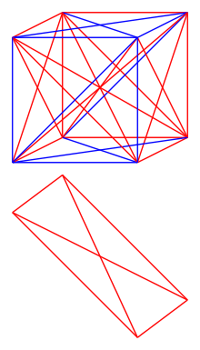Graham's number $$G_{64}$$ is a famous large number, defined by Ronald Graham.

Using Up-arrow notation, it is defined as the 64th term of the following sequence:

$$\begin{array}{l} G_0=4 \\ G_1=3\uparrow\uparrow\uparrow\uparrow3 \\ \textrm{For}\;0\le k<64\textrm{,}\;G_{k+1}=3\underbrace{\uparrow\uparrow\cdots\uparrow\uparrow}_{G_k}3 \\ \text{Graham's number}=G_{64}\end{array}$$

($$G_n$$ is sometimes written $$G(n)$$, $$g_n$$ or $$g(n)$$)

Graham's number is commonly celebrated as the largest number ever used in a serious mathematical proof, although much larger numbers have since claimed this title (such as TREE(3) and SCG(13)). The smallest Bowersism exceeding Graham's number is Corporal, and the smallest Saibianism exceeding Graham's number is Graatagold.

## History

Graham's number arose out of the following unsolved problem in Ramsey theory:

Let N* be the smallest dimension n of a hypercube such that if the lines joining all pairs of corners are two-colored for any nN*, a complete graph K4 of one color with coplanar vertices will be forced. Find N*.An example of a cube with 12 planar K4's, with a single monochromatic K4 shown below. If you change the edge on the bottom of this K4 to blue, then the cube will contain no monochromatic planar K4's, thus showing that N* is at least 4.

To understand what this problem asks, first consider a hypercube of any number of dimensions (1 dimension would be a line, 2 would be a square, 3 would be a cube, 4 would be a tesseract (4-dimensional cube), etc.), and call that number of dimensions N. Then, imagine connecting all possible vertex pairs with lines, and coloring each of those red or blue - one such way you can color all of the vertex pairs of the 3-dimensional cube is shown to the right. What is the smallest number of dimensions N such that all possible colorings would have a monochromatic complete graph of four coplanar vertices (that is a set of four points that are connected in all possible ways, with all lines being the same color)?

Graham published a paper in 1971 proving that the answer exists, providing the upper bound $$F^{7}(12)$$, where $$F(n) = 2 \uparrow^{n} 3$$ in Arrow notation. Sbiis Saibian calls this number "Little Graham". Martin Gardner, when discovering the number's size, found it difficult to explain, and he devised a larger, easier-to-explain number which Graham proved in an unpublished 1977 paper. Martin Gardner wrote about the number in Scientific American and it even made it to Guiness World Records in 1980 as the largest number used in a mathematical proof, although a few years later the title was removed from Guinness World Records.

In 2013, the upper bound was further reduced to N' = 2↑↑2↑↑(3+2↑↑8) using the Hales–Jewett theorem , and to N" = (2↑↑5138) x ((2↑↑5140)↑↑(2 x (2↑↑5137))) << 2↑↑↑5 in 2019 . As of 2014, the best known lower bound for N* is 13, shown by Jerome Barkley in 2008.

## Comparison

Since g0 is 4 and not 3, Graham's number cannot be expressed efficiently in Chained arrow notation $$g_{64} \approx 3 \rightarrow 3 \rightarrow 64 \rightarrow 2$$ or BEAF $$\{3,65,1,2\} < g_{64} < \{3, 66, 1, 2\}$$. Using Jonathan Bowers' base-3 G functions it is exactly G644. It can be also exactly expressed in the Graham Array Notation as $$[3,3,4,64]$$.

Tim Chow proved that Graham's number is much larger than the Moser. The proof hinges on the fact that, using Steinhaus-Moser Notation, n in a (k + 2)-gon is less than $$n\underbrace{\uparrow\uparrow\ldots\uparrow\uparrow}_{2k-1}n$$. He sent the proof to Susan Stepney on July 7, 1998. Coincidentally, Stepney was sent a similar proof by Todd Cesere several days later.

It has been proven that Graham's number is much less than $$\Sigma(64)$$. According to Wythagoras, he found a Turing machine that proves $$\Sigma(18) > G$$ by improving Deedlit11's machine, and wrote a brief sketch of the proof instead of a rigorous proof. In this community, it was believed that a better upper bound $$\Sigma(17)$$ was proven by someone until 09/07/2021, but at least there was no cited source about the result. Therefore the reader should be careful that he or she might have caught a wrong information.

Japanese googologist Fish proved that Graham's number is less than $$f_{\omega+1}(64)$$ in the Fast-growing hierarchy with respect to Wainer hierarchy.

## Approximations in other notations

Notation Approximation
Fast-growing hierarchy $$f_{\omega+1}(64)$$
Chained arrow notation $$3 \rightarrow 3\rightarrow 64\rightarrow 2$$
Hardy hierarchy $$H_{\omega^\omega 64}(4)$$
BEAF $$\{3,65,1,2\}$$
Extended Hyper-E Notation $$\textrm E3\#\#4\#64$$
Strong array notation $$s(3,64,2,2)$$
Slow-growing hierarchy $$g_{\Gamma_0}(65)$$

## Calculating last digits

The final digits of Graham's number are not easily computed by a simple application of algorithms for modular exponentiation. Here is a simple but wrong algorithm to obtain the last $$x$$ digits $$N(x)$$ of Graham's number:

• $$N(0) = 3$$
• $$N(x) = 3^{N(x-1)} \text{ mod } 10^x$$

Assume that this method works. Then for any positive integer $$x$$ such that $$G_{64} < 10^x$$, $$N(x)$$ coincides with $$G_{64}$$. It means that $$3^{G_{64}} - G_{64}$$ is divisible by $$10^x$$ for any positive integer $$x$$. It implies $$3^{G_{64}} - G_{64} = 0$$, and it is a contradiction.

It is natural to ask the maximum of positive integer $$x$$ such that the computation above outputs the correct value of $$N(x)$$. See the main article on modular exponentiation.

While it is not known how to calculate the leading digit of Graham's number in base 10 in the current community, the leading digit must be 1 in base 2 (because all positive integers except 0 have this property), 1 in base 3 (because it is a power of 3) , and 3 in base 9 (because it is an odd-numbered power of 3). It is not known how to calculate the leading digit of Graham's number in any other base in the current community, unless if it is a power of 3 (such as 27), or at least comparable to Graham's number (such as Graham's number - 64).